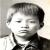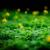## 还是二叉树的插入、删除子树问题

```#include <stdio.h>
#include <stdlib.h>
typedef struct bitnode
{
char data;
struct bitnode *lchild, *rchild;
} BiTNode, *BiTree;
BiTree Initiate();
BiTree Insert_L(BiTree bt, char x, BiTree parent);
void PreOrder_0(BiTree bt);
void CreateBinTree(BiTree *T);
void PreOrder(BiTree bt);
int i,n;
BiTree parent;
BiTree Initiate()                           //带头结点的空二叉树
{
BiTNode *bt;
bt=(BiTNode*)malloc(sizeof(BiTNode));
bt->lchild=NULL;
bt->rchild=NULL;
return bt;
}
BiTree Insert_L(BiTree bt, char x, BiTree parent)
{
BiTree p;
if(parent==NULL)
{
printf("\n插入错误");
return NULL;
}
if((p=(BiTNode*)malloc(sizeof(BiTNode)))==NULL)
return NULL;
p->data=x;
p->lchild=NULL;
p->rchild=NULL;
if(parent->lchild==NULL)
parent->lchild=p;
else
{
p->lchild=parent->lchild;
parent->lchild=p;
}
return bt;
}
void PreOrder_0(BiTree bt)        //查找parent函数
{
i++;
if(bt==NULL)
return;
if(i==n)                     //关键是这里，明明设置了结束子程序条件，可就是对右子树无效。
{
parent=bt;
return;
}
PreOrder_0(bt->lchild);
PreOrder_0(bt->rchild);
}
void CreateBinTree(BiTree *T)
{
char a;
scanf("%c",&a);
getchar();
if(a=='0')
(*T)=NULL;
else
{
(*T)=(BiTNode*)malloc(sizeof(BiTNode));
(*T)->data=a;
CreateBinTree(&((*T)->lchild));
CreateBinTree(&((*T)->rchild));
}
}
void PreOrder(BiTree bt)
{
if(bt==NULL)
return;
printf("%c ",bt->data);
PreOrder(bt->lchild);
PreOrder(bt->rchild);
}
void main()
{
char x;
BiTree bt;
bt=Initiate();
if(bt)
{
printf("请输入二叉树元素:");
CreateBinTree(&bt);
printf("二叉树的先序遍历为:");
PreOrder(bt);
printf("\n");
printf("输入要作为父节点所在的位置:");
scanf("%d",&n);
i=0;
PreOrder_0(bt);
getchar();
printf("输入要插入的元素:");
scanf("%c",&x);
Insert_L(bt, x, parent);
PreOrder(bt);
printf("\n");
}
} ```

### 以下是问题补充：

@剑麟：void PreOrder_0(BiTree bt) { i++; if(bt==NULL) return; if(i==n) //关键是这里，明明设置了结束子程序条件，可就是对右子树无效。 { parent=bt; return; } PreOrder_0(bt->lchild); PreOrder_0(bt->rchild); } 关键是这部分。比如一颗二叉树的先序：ABDCEF,中序：DBAECF；我的程序是想，根据先序的顺序，输入要作为父节点的元素在先序中的位置，然后用PreOrder_0() 函数来找出这个元素。 例如我要把B作为parent，它在先序中的位置为n=2，然后用 i 来计算循环次数，从而找出parent。 可是不知为什么，对C\E\F 这种元素却没效，很是头疼！ (2012/05/01 22:39)

0000A B D 0 0 0 C E 0 0 F 0 0 按照你的逻辑，会这样存放。 A，B，D会存放在BT的左侧叶子，A在BT自身。但你 000三个了。所以会跳到 BT的兄弟结点上，开始折腾后面的。所以你在BT结点下面查找自然找不到。我的输入，和你的输入不一样，不要说，你也是这样输入的。。。。